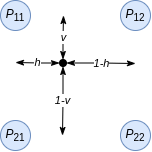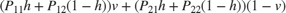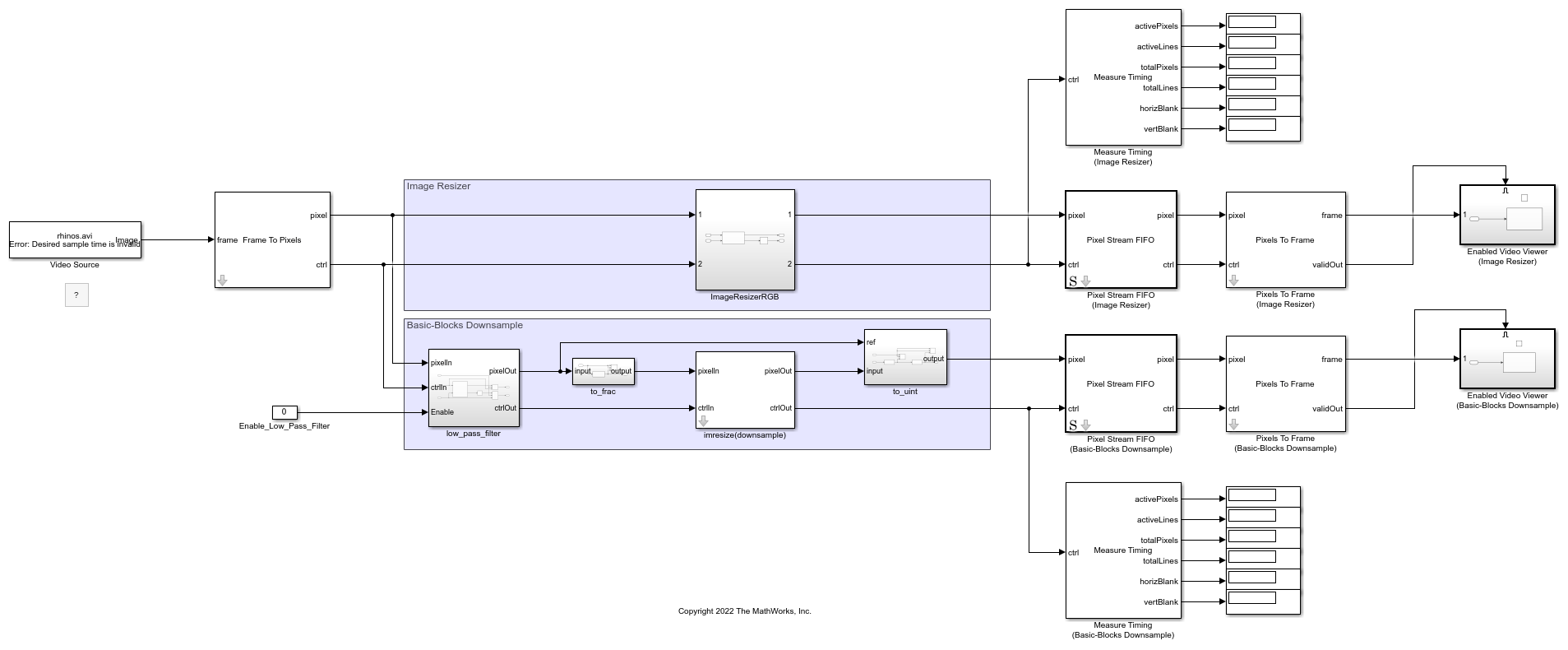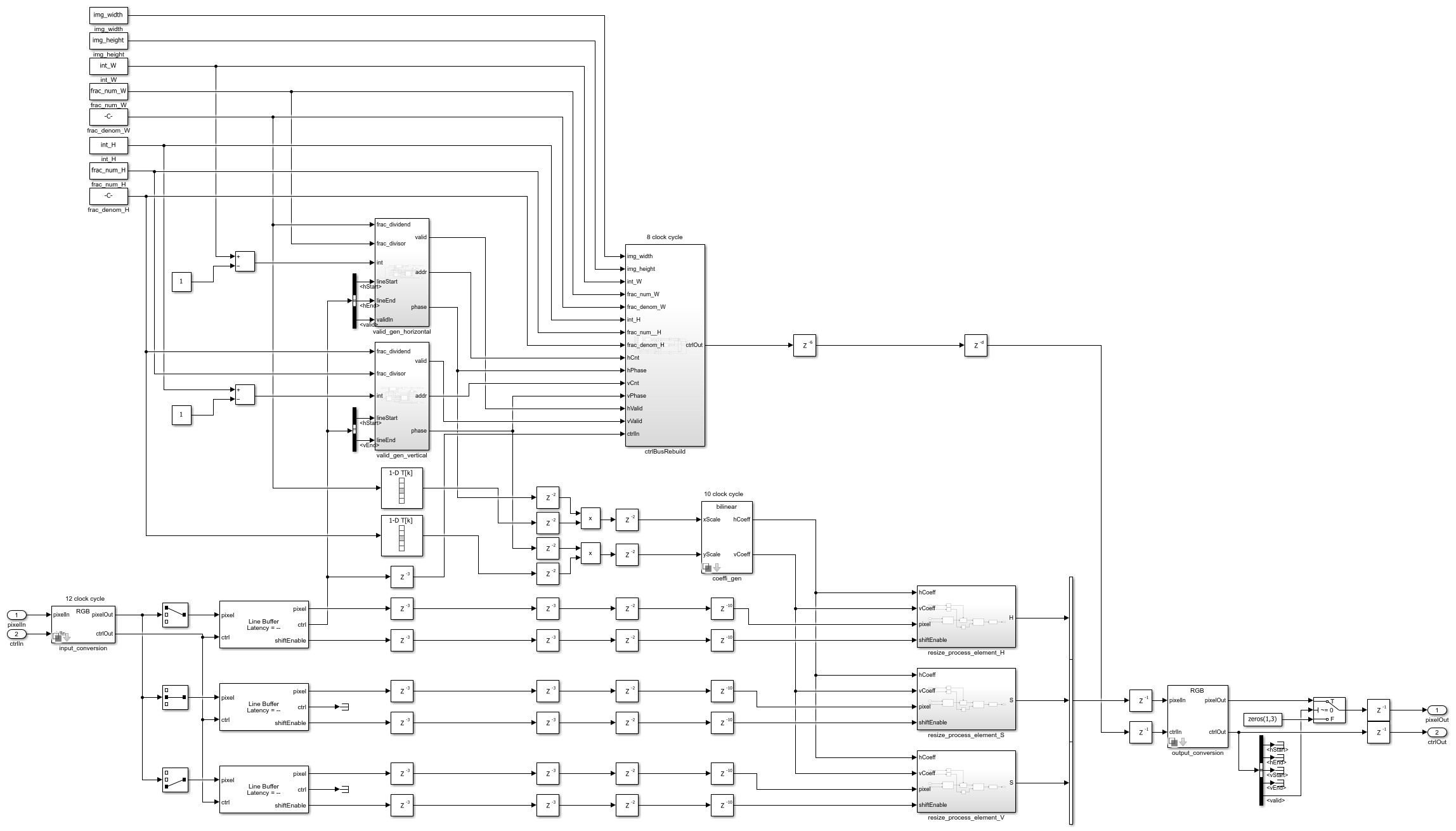# Image Resize

This example shows how to downsample an image by using the bilinear, bicubic, or Lanczos-2 interpolation algorithm. The implementation uses an architecture suitable for FPGAs.

In theory, you can achieve exact image reconstruction by resizing the image using a sinc kernel. However, sinc kernels have infinite spatial extent. To limit the extent, interpolation implementations use simpler kernels to approximate a sinc. The bilinear interpolation algorithm uses the weighted sum of the nearest four pixels to determine the values of the output pixels. Bicubic and Lanczos-2 interpolations are approximations of a sinc kernel. Bicubic interpolation is a more computationally efficient version of the Lanczos-2 method.

### Behavioral Reference

By default, the Image Processing Toolbox™ function imresize uses the bicubic interpolation algorithm. You can choose bilinear or Lanczos-2 interpolation algorithms by setting the 'Method' name-value pair argument to 'bilinear' or 'lanczos-2', respectively.

v = VideoReader('rhinos.avi'); I = readFrame(v); Y = imresize(I,[160,256],'Method','bilinear'); figure; imshow(Y);### Interpolation Algorithms

Bilinear interpolation determines the inserted pixel value from the weighted average of the four input pixels nearest to the inserted location.andare horizontal scale and vertical scale, respectively, and are calculated independently.The value for each output pixel is given by.

The bicubic algorithm calculates the average of the 16 input pixels nearest to the inserted location.The bicubic coefficients are given by.

These equations show that the bilinear and bicubic algorithms calculate coefficients for each output pixel.

The Lanczos-2 algorithm precalculates the coefficients based on the resize factor. The model calls the lanczos2_coeffi.m script to calculate and store these coefficients. The script calculates the Lanczos-2 coefficients using 6 taps and 32 phases.

### Implementation of Interpolation Algorithms for HDL

This figure shows the principle used to implement the image resize algorithm for hardware. For example, consider downsampling an image by a scale factor of 3/4. One possible implementation of downsampling an image by 3/4 is to upsample by a factor of 3 and then downsample by a factor of 4. The figure shows the pixel indexes after these operations. Blue dots represent the original pixels, and green crosses represent the interpolated pixels after upsampling.The indexes after downsampling show that not all the interpolated pixels are used in the output image. This example implements a more efficient version of the downsample step by generating interpolated pixels only when they are needed in the output image.

The phase, shown in the bottom line of the figure, is an index that selects which pixels are needed for the output image. When the phase is 0, the algorithm returns the original input pixel value. When the phase is 1, the algorithm calculates coefficients to generate the interpolated pixel in the first position. When the phase is 2, the algorithm calculates coefficients to generate the interpolated pixel in the second position.

### Example Model

Similar to the imresize function, the imresize(downsample) subsystem in this model supports two ways to define the output image size. You can specify a scale factor ranging from 1.000 to 127.999, or you can define the output frame width and height in pixels. Double-click the imresize(downsample) subsystem to set its parameters.

To avoid aliasing introduced by lowering the sampling rate, the model includes a lowpass filter before the imresize(downsample) subsystem. After the imresize(downsample) subsystem, the Pixel Stream FIFO block consolidates the output pixels into contiguous lines of valid pixels surrounded by blanking intervals of invalid pixels. This FIFO is optional. Use the FIFO when you want to take advantage of the increased blanking interval to perform further computations on the pixel stream. The Measure Timing block displays the size of the output frames.

modelname = 'ImageResizeForFPGAHDL'; open_system(modelname); set_param(modelname,'SampleTimeColors','on'); set_param(modelname,'Open','on'); set(allchild(0),'Visible','off');In the imresize(downsample) subsystem, the input_conversion and output_conversion subsystems convert the colorspace of the pixel stream based on the parameter on the mask. The valid_gen_horizontal and valid_gen_vertical subsystems return control signals that are used for generating coefficients and rebuilding the output control bus. If the last line of the image contains no valid pixels after downsampling, the ctrlBusRebuild subsystem rebuilds the control bus for the new size.

open_system([modelname '/imresize(downsample)'],'force');This diagram shows the expected output from the valid_gen_horizontal and valid_gen_vertical subsystems. The valid signal indicates the validity of the current address and the corresponding phase. To simplify rebuilding the control bus, the first line and row of each output frame are always valid.The coefficient generation subsystem, coeffi_gen, is a variant subsystem, where bilinear, bicubic, and Lanczos-2 coefficient generators are implemented separately. You can select the algorithm from the mask.

open_system([modelname '/imresize(downsample)/coeffi_gen'],'force');The resize_process_element subsystems multiply the coefficients with each pixel component by using a separable filter in vertical order and then in horizontal order. The trim_0_1 subsystem ensures the result is between 0 and 1.

open_system([modelname '/imresize(downsample)/resize_process_element_H'],'force');### Resource Usage

These tables show the resource usage for the imresize(downsample) subsystem with 240p video input, and do not include the lowpass filter or the Pixel Stream FIFO. The design was synthesized to achieve a clock frequency of 150 MHz.

This table shows the resources for each of the three algorithms when downsampled in the HSV colorspace.This table shows the resources for each of the three algorithms when downsampled in the RGB colorspace.### References

 Keys, R. "Cubic Convolution Interpolation for Digital Image Processing." IEEE Transactions on Acoustics, Speech, and Signal Processing 29, no. 6 (December 1981): 1153-60. https://doi.org/10.1109/TASSP.1981.1163711.

 Smith, Alvy Ray. "Color Gamut Transform Pairs." In Proceedings of the 5th Annual Conference on Computer Graphics and Interactive Techniques - SIGGRAPH '78, 12-19. Not Known: ACM Press, 1978. https://doi.org/10.1145/800248.807361.

## SupportGet trial now